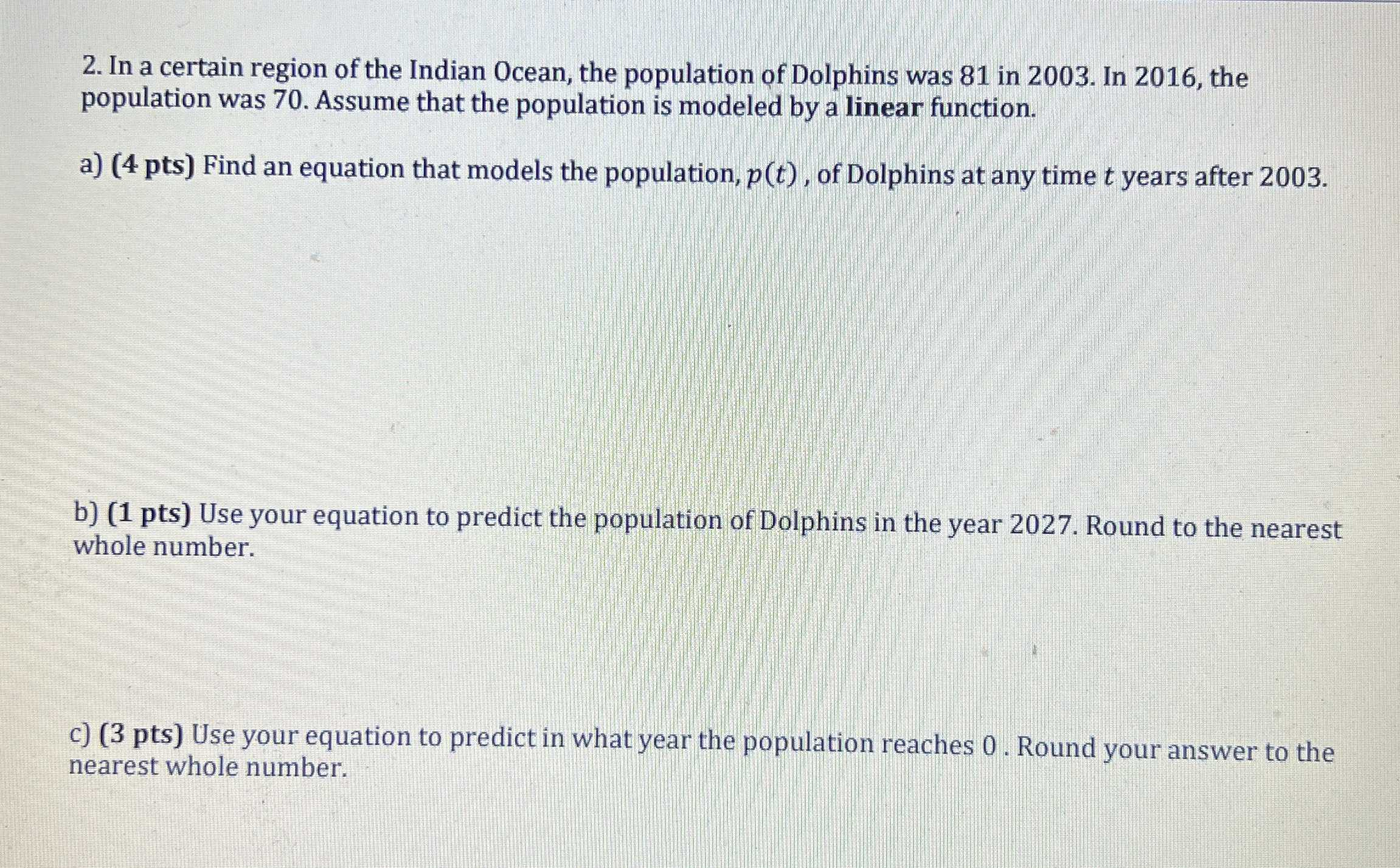### ¿Todavía tienes preguntas de matemáticas?

Pregunte a nuestros tutores expertos
Algebra
Pregunta2. In a certain region of the Indian Ocean, the population of Dolphins was $$81$$ in $$2003 .$$ In $$2016$$ , the population was $$70$$ . Assume that the population is modeled by a linear function. a) ( $$4$$ pts) Find an equation that models the population, $$p ( t )$$ , of Dolphins at any time $$t$$ years after $$2003$$ . b) (1 pts) Use your equation to predict the population of Dolphins in the year $$2027$$ . Round to the nearest whole number. c) ( $$3$$ pts) Use your equation to predict in what year the population reaches $$0$$ . Round your answer to the nearest whole number.

a)

if we take 2003 as t = 0, then,

slope = (70 - 81) / (13 - 0) = -11/13

y-intercept = p(0) = 81

So, the equation of the line is, p(t) = -(11/13)t + 81

b)

if the year is 2027, then t = 2027 - 2003 = 24

So, p(24) = -(11/13)24 + 81 = 61

c)

That is p(t) = 0,

So,

-(11/13)t + 81 = 0

(11/13)t = 81

t = (13/11)×81 = 96 years after 2003.

That is in the year = 2003 + 96 = 2099.

Solución
View full explanation on CameraMath App.